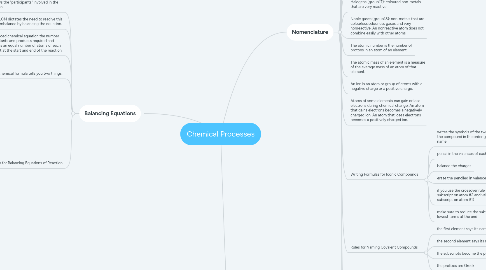# Chemical Processes

Get Started. It's FreeChemical Processes## 1. Balancing Equations

### 1.4. A chemical formula tells you two things:

1.4.1. What kinds of atoms there are in a compound

1.4.2. How many of each atom there is in a compound.

### 1.5. Tips for Balancing Equations of Reaction

1.5.1. Write a word equation and convert it to a skeletal equation

1.5.2. Write the reactants and the products using proper chemical notation.

1.5.3. Place a box around all of the formulas in your equation so you are not tempted to change them. You are not allowed to change the subscripts of a formula - the formula is the formula. You are only allowed to change coefficients - the numbers that go in front of the formula.

1.5.4. Make yourself a balance sheet.

1.5.5. Balance the atoms other than H and O first.

1.5.6. Balance H atoms next.

1.5.7. Balance O atoms last.

1.5.8. If you have polyatomic ions that are the same on both sides, balance them as a group.

## 2. Nomenclature

### 2.30. Writing Formulas for Iconic Compounds

2.30.1. writes the symbols of the two elements in the compound in the order given in the name

2.30.2. pencil in the valences of each

2.30.3. balance the charges

2.30.4. erase the penciled in valences

2.30.5. if you use the crossover rule (valence #1 = subscript on atom #2 and valence #2 = subscript on atom #1)

2.30.6. make sure to reduce the subscripts to their lowest terms at the end

### 2.31. Rules for Naming Covalent Compounds

2.31.1. the first element says its name

2.31.2. the second element says its name

2.31.3. the subscripts become the prefixes

2.31.4. the prefixes are Greek

### 2.32. Greek Prefixes (prefix - number of atoms)

2.32.1. mono - 1

2.32.2. di - 2

2.32.3. tri - 3

2.32.4. tetra - 4

2.32.5. penta - 5

2.32.6. hexa - 6

2.32.7. hepta - 7

2.32.8. octa - 8

2.32.9. nona - 9

2.32.10. deca - 10

### 2.33. Writing Formulas for Covalent Compounds

2.33.1. write the symbols of the two elements in the compound in the order given in the name

2.33.2. the prefix = the subscript for the atom

2.33.3. Please note that for covalent compounds you do not reduce the subscripts to lowest terms at the end

### 2.34. Rules for Naming Oxy Acids

2.34.1. name the element after the hydrogen

2.34.2. take the root of the name and add “ic” to it

2.34.3. add acid to the name

### 2.35. Writing Acid Formulas Summary

2.35.1. Binary Acid

2.35.1.1. hydro____ic acid H non-metal atomic symbol (balance the charges)

2.35.2. Oxy Acid

2.35.2.1. ____ic acid H non- metal atomic symbol O (consult your chart for subscripts)

### 2.36. Rules for Naming Binary Acids

2.36.1. the first element, or hydrogen part of the name is “hydro”

2.36.2. the second element ending changes to “ic”

2.36.3. the name is always followed by the word “acid”

### 2.37. Rules for Writing the Formulas for Binary Acids

2.37.1. write the atomic symbols for the elements in the acid

2.37.2. pencil in the charges up top

2.37.3. using the crisscross rule, balance the charges by putting in subscripts as needed

2.37.4. erase the charges

### 2.38. Naming Oxy Acid Salts Summary

2.38.1. oxy acid salt (metal+polyatomic non-metal)

2.38.1.1. metal states its name

2.38.1.2. polyatomic non-metal states its name

### 2.39. Transition Metal

2.39.1. metal states its name

2.39.2. roman numeral used to indicate charge on the metal ion

2.39.3. polyatomic non-metal states its name

## 3. The Chemical Reaction

### 3.1. A Chemical Change = A Chemical Reaction

3.1.1. A chemical reaction occurs when a substance or substances change into one or more different substances. You can tell a chemical reaction has occurred if you observe one or more of the following:

3.1.1.1. an unexpected colour change

3.1.1.2. the production of heat, light or sound&nbsp;

3.1.1.3. the appearance or disappearance of an odour&nbsp;

3.1.1.4. the formation of a precipitate&nbsp;

3.1.1.5. the formation of a gas

### 3.4. Five common types of chemical reactions are:

3.4.1. Synthesis

3.4.1.1. A+B→AB

3.4.2. Decomposition

3.4.2.1. AB→A+B

3.4.3. Single Displacement

3.4.3.1. AB+C→CB+A

3.4.4. Double Displacement